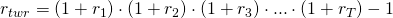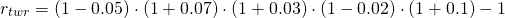# Time-weighted return (TWR)

The time-weighted return (TWR) or time-weighted rate of return (TWRR) of an investment is the buy-and-hold return that and investors will earn when they have bought the fund and held it passively over time. It differs from the dollar-weighted or money-weighted return, where we also take into consideration investors withdrawals and deposits over the life of the investment.

Time-weighted returns do not take into account the timing of inflows into and outflows out of the portfolio. Since fund managers, in general, do not have any control over the flows in and out of a portfolio, time-weighted rate of returns are an appropriate measure for performance in the context of mutual funds and other types of funds. They provide a measure of performance that is not influenced by the decisions made by investors (i.e. adding and withdrawing money). Now, let’s go over to how to calculate time-weighted returns.

## time weighted return formula

The time-weighted rate of return is fairly simple and is very similar to calculating the compounded rate of return.The time-weighted rate of return formula is the following## Time-weighted rate of return example

Let’s consider a simple example that applies the above formula to a set of returns. Suppose we have a mutual fund manager that reports the following set of annual returns over the past 5 years; -5%, 7%, 3% – 2%, and 10%. Now we want to know what an investment at the start of this 5-year period would have yielded. To do this, we apply the above formulawhich equals 12.87%. This means that over a 5-year period, an investor will have earned a cumulative return of 12.87%. When we annualize this return, we find that the investor earned 2.5% per year, on average.

## Summary

Time-weighted returns are very useful when analyzing the buy-and-hold return that an investor would have earned. We when we want to have a good measure of the actual performance, however, it is better to use dollar-weighted returns.

### Time weighted return calculator

Want calculate TWR returns yourself? Download the time weighted return calculator: time-weighted return